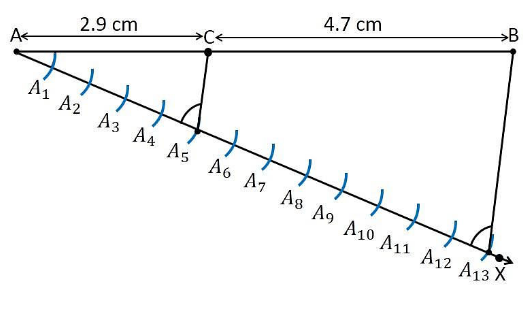# Draw a line segment of length 7.6 cm and divide it in the ratio 5 : 8. Measure the two parts. Give the justification of the construction

Procedure

A line segment with a measure of 7.6 cm length is divided in the ratio of 5:8 as follows.

1. Draw line segment AB with the length dimension of 7.6 cm

2. Draw a ray AX that shapes an acute angle with line segment AB.

3. Position the points i.e.,13 (= 5+8) points, such as A1, A2, A3, A4 …….. A13, on the ray AX such that it becomes AA1 = A1A2 = A2A3 and so on.

4. Join the line segment and the ray, BA13.

5. Now through the point A5, draw a line parallel to BA13 which makes an angle equal to ∠AA13B

6. The point A5 intersects the line AB at point C.

7The point C divides line segment AB of 7.6 cm in the required ratio of 5:8.

8. Now, mark the lengths of the line AC and CB. It comes out to the measure of 2.9 cm and 4.7 cm respectively.Justification:

AC/CB = 5/ 8

By construction, we have

A5C || A13B.

From Basic proportionality theorem for the triangle AA13B, we get

AC/CB =AA5/A5A13….. (1)

From the diagram, we can obseerve that AA5 and A5A13 contain 5 and 8 equal divisions of line segments respectively.

Therefore, it becomes

AA5/A5A13=5/8… (2)

On comparing the equations (1) and (2), we get

AC/CB = 5/ 8

Hence, Justified.